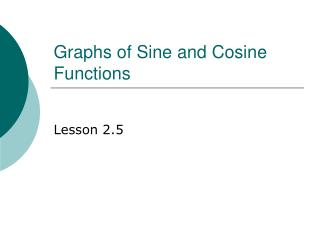DownloadDownload PresentationGraphs of Sine and Cosine Functions

# Graphs of Sine and Cosine Functions

Télécharger la présentation## Graphs of Sine and Cosine Functions

- - - - - - - - - - - - - - - - - - - - - - - - - - - E N D - - - - - - - - - - - - - - - - - - - - - - - - - - -
##### Presentation Transcript

1. Graphs of Sine and Cosine Functions Lesson 2.5

2. Ordered Pairs • Consider the values for x and y in the table to the right • Note • Period = 2π • Maximum y values • Minimum y values

3. Period = 2π Maximum and minimum values Graphing the Ordered Pairs

4. Graphing on Calculator • Go to ♦Y= screen • Enter function • Choose F2, zoom 7-Trig • Graph is plotted • Tic marks are inunits of π/2 Try Web Graphing Utility

5. amplitude = 1 Amplitude • Defined as the absolute value of maximum or minimum of the function • Try graphingy = 2 cos x • What is the amplitude • For y = a cos x or y = a sin x • The amplitude is |a|

6. Period of a Trig Function(Recall slide from previous lesson) • The functions repeat themselves • The period is the smallest value, p for which f(x) = f(x + p) • For sin, cos, sec, csc • The period is 2π • For tan and ctn • The period is π

7. Period of a Trig Function • What happens for ? • Try graphing y = sin 3x • What is the period? • Try y = cos 0.5x • What is the period? • For • Period = Same for cos, sec, csc

8. Period of a Trig Function • For tangent • Note amplitudeis without bound • Period is π • For • Period = • Predict the period fory = tan (1/3 x) • Graph it and verify your prediction

9. Assignment • Lesson 2.5 • Page 177 • Exercises 1 – 61 EOO also 63